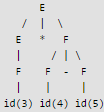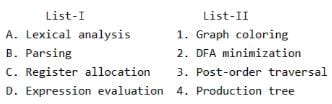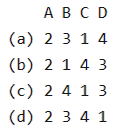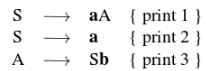# Test: Parsing- 2

## 20 Questions MCQ Test RRB JE for Computer Science Engineering | Test: Parsing- 2

Description
Attempt Test: Parsing- 2 | 20 questions in 60 minutes | Mock test for Computer Science Engineering (CSE) preparation | Free important questions MCQ to study RRB JE for Computer Science Engineering for Computer Science Engineering (CSE) Exam | Download free PDF with solutions
QUESTION: 1

### Consider the following expression grammar. The seman­tic rules for expression calculation are stated next to each grammar production. E → number E.val = number. val | E '+' E E(1).val = E(2).val + E(3).val | E '×' E E(1).val = E(2).val × E(3).val   Q. The above grammar and the semantic rules are fed to a yacc tool (which is an LALR (1) parser generator) for parsing and evaluating arithmetic expressions. Which one of the following is true about the action of yacc for the given grammar?

Solution:

Background yacc conflict resolution is done using following rules: shift is preferred over reduce while shift/reduce conflict. first reduce is preferred over others while reduce/reduce conflict.   You can answer to this question straightforward by constructing LALR(1) parse table, though its a time taking process. To answer it faster, one can see intuitively that this grammar will have a shift-reduce conflict for sure. In that case, given this is a single choice question, (C) option will be the right answer. Fool-proof explanation would be to generate LALR(1) parse table, which is a lengthy process. Once we have the parse table with us, we can clearly see that i. reduce/reduce conflict will not arise in the above given grammar ii. shift/reduce conflict will be resolved by giving preference to shift, hence making the expression calculator right associative. According to the above conclusions, only correct option seems to be (C).

QUESTION: 2

### Consider the following expression grammar. The seman­tic rules for expression calculation are stated next to each grammar production. E → number E.val = number. val | E '+' E E(1).val = E(2).val + E(3).val | E '×' E E(1).val = E(2).val × E(3).val   Q. Assume the conflicts in Part (a) of this question are resolved and an LALR(1) parser is generated for parsing arithmetic expressions as per the given grammar. Consider an expression 3 × 2 + 1. What precedence and associativity properties does the generated parser realize?

Solution:

Answer is B as the productions belong to the same non-terminal and since YACC resolves by shift over reduce, the associativity will be right associative.

QUESTION: 3

### Which of the following grammar rules violate the requirements of an operator grammar ? P, Q, R are nonterminals, and r, s, t are terminals. 1. P → Q R 2. P → Q s R 3. P → ε 4. P → Q t R r

Solution:
QUESTION: 4

Consider the grammar with the following translation rules and E as the start symbol.

E → E1 # T {E.value = E1.value * T.value}
| T{E.value = T.value}
T → T1 & F {T.value = T1.value + F.value}
| F{T.value = F.value}
F → num {F.value = num.value}

Q. Compute E.value for the root of the parse tree for the expression: 2 # 3 & 5 # 6 & 4.

Solution:
QUESTION: 5

Which of the following suffices to convert an arbitrary CFG to an LL(1) grammar?

Solution:

Removing left recursion and factoring the grammar do not suffice to convert an arbitrary CFG to LL(1) grammar.

QUESTION: 6

Assume that the SLR parser for a grammar G has n1 states and the LALR parser for G has n2 states. The relationship between n1 and n2 is:

Solution:
QUESTION: 7

In a bottom-up evaluation of a syntax directed definition, inherited attributes can

Solution:

A Syntax Directed Definition (SDD) is called S Attributed if it has only synthesized attributes. L-Attributed Definitions contain both synthesized and inherited attributes but do not need to build a dependency graph to evaluate them.

QUESTION: 8

Consider the grammar shown below S → i E t S S' | a S' → e S | ε E → b In the predictive parse table. M, of this grammar, the entries M[S', e] and M[S', \$] respectively are

Solution:

Here representing the parsing table as M[ X , Y], where X represents rows(Non terminals) and Y represents columns(terminals). Here are the rules to fill the parsing table. For each distinct production rule A->α, of the grammar, we need to apply the given rules:
Rule 1: if A –> α is a production, for each terminal ‘a’ in FIRST(α), add A–>α to M[ A , a]
Rule 2 : if ‘ ε ‘ is in FIRST(α), add A –> α to M [A , b] for each ‘b’ in FOLLOW(A). As Entries have been asked corresponding to Non-Terminal S', hence we only need to consider its productions to get the answer. For S' → eS, according to rule 1, this production rule should be placed at the entry M[ S', FIRST(eS)], and from the given grammar, FIRST(eS) ={e}, hence S'->eS is placed in the parsing table at entry M[S' , e]. Similarly, For S'->ε, as FIRST(ε) = {ε}, hence rule 2 should be applied, therefore, this production rule should be placed in the parsing table at entry M[S',FOLLOW(S')], and FOLLOW(S') = FOLLOW(S) = {e, \$}, hence R->ε is placed at entry M[S', e] and M[S' , \$]. Therefore Answer is option D. Visit the Following links to Learn how to find First and Follow sets.

QUESTION: 9

Consider the grammar shown below.

S → C C
C → c C | d

The grammar is

Solution:

Since there is no conflict, the grammar is LL(1). We can construct a predictive parse table with no conflicts. This grammar also LR(0), SLR(1), CLR(1) and LALR(1).

QUESTION: 10

Consider the translation scheme shown below

S → T R
R → + T {print ('+');} R | ε
T → num {print (num.val);}

Here num is a token that represents an integer and num.val represents the corresponding integer value. For an input string '9 + 5 + 2', this translation scheme will print

Solution:

Let us make the parse tree for 9+5+2 in top down manner, left first derivation.

Steps:
1) Exapnd S->TR
2) apply T->Num...
3) apply R -> +T...
4) appy T->Num...
5) apply R-> +T..
6) apply T-> Num..
7) apply R-> epsilon

After printing through the print statement in the parse tree formed you will get the answer as 95+2+

QUESTION: 11

Consider the syntax directed definition shown below.

S → id : = E {gen (id.place = E.place;);}
E → E1 + E2 {t = newtemp ( ); gen (t = El.place + E2.place;); E.place = t}
E → id {E.place = id.place;}

Here, gen is a function that generates the output code, and newtemp is a function that returns the name of a new temporary variable on every call. Assume that ti's are the temporary variable names generated by newtemp. For the statement 'X: = Y + Z', the 3-address code sequence generated by this definition is

Solution:

It must be B. The production E --> E + E is used only one time and hence only one temporary variable is generated.

QUESTION: 12

Which of the following statements is false?

Solution:

1. A grammar is ambiguous if there exists a string s such that the grammar has more than one leftmost derivations for s. We could also come up with more than one rightmost derivations for a string to prove the above proposition, but not both of right and leftmost. An unambiguous grammar can have different rightmost and leftmost derivations.
2. LL parser is top-down by nature. Leftmost derivation is, intuitively, expanding or top-down in fashion, hence such convention. Rightmost derivation, on the other hand, seems like a compressing or bottom-up thing.
3. LALR is more powerful than SLR, even when both have the same LR(0) states, due to the fact that SLR checks for lookaheads by looking at FIRST and FOLLOW from the grammar after constructing its parse table and on the other hand, LALR computes lookaheads from the LR(0) states while constructing the parse table, which is a better method.
4. An ambiguous grammar can never be LR(k) for any k, because LR(k) algorithm aren’t designed to handle ambiguous grammars. It would get stuck into undecidability problem, if employed upon an ambiguous grammar, no matter how large the constant k is

QUESTION: 13

Which of the following derivations does a top-down parser use while parsing an input string? The input is assumed to be scanned in left to right order.

Solution:
QUESTION: 14

Given the following expression grammar:

E -> E * F | F + E | F
F -> F - F | id

which of the following is true? (GATE CS 2000)

Solution:

Let say i/p is 3*4-5 when we draw parse tree according to grammarAs we can see first '- ' will be evaluated then ' * ' is evaluated so ' - ' has higher precedence then *. So correct choice is B See question 1 of

QUESTION: 15

Which one of the following is True at any valid state in shift-reduce parsing?

Solution:

The prefixes of right sentential forms that can appear on the stack of a shift-reduce parser are called viable prefixes. By definition, a viable prefix is a prefix of a right sentential form that does not continue past the right end of the rightmost handle of that sentential form.

QUESTION: 16

In the context of abstract-syntax-tree (AST) and control-flow-graph (CFG), which one of the following is True?

Solution:

(A) is false, In CFG (Control Flow Graph), code of N2 may be present before N1 when there is a loop or goto.
(B) is false, CFG (Control Flow Graph) contains cycle when input program has loop.
(C) is true, successors in AST and CFG depedend on input program
(D) is false, a single statement may belong to a block of statements.

QUESTION: 17

Match the following:Codes:Solution:

Register allocation is a variation of Graph Coloring problem.
Lexical Analysis uses DFA.
Parsing makes production tree Expression evaluation is done using tree traversal

QUESTION: 18

Among simple LR (SLR), canonical LR, and look-ahead LR (LALR), which of the following pairs identify the method that is very easy to implement and the method that is the most powerful, in that order?

Solution:

SLR parser is a type of LR parser with small parse tables and a relatively simple parser generator algorithm. Canonical LR parser or LR(1) parser is an LR(k) parser for k=1, i.e. with a single lookahead terminal. It can handle all deterministic context-free languages.LALR parser or Look-Ahead LR parser is a simplified version of a canonical LR parser,

QUESTION: 19

Consider the following grammar G.

S → F ⎪ H
F → p ⎪ c
H → d ⎪ c

Q. Where S, F and H are non-terminal symbols, p, d and c are terminal symbols. Which of the following statement(s) is/are correct?

S1: LL(1) can parse all strings that are generated using grammar G.
S2: LR(1) can parse all strings that are generated using grammar G.

Solution:

The given grammar is ambiguous as there are two possible leftmost derivations for string "c".

First Leftmost Derivation
S → F
F → c

Second Leftmost Derivation
S → H
H → c

An Ambiguous grammar can neither be LL(1) nor LR(1)

QUESTION: 20

Consider the following Syntax Directed Translation Scheme (SDTS), with non-terminals {S, A} and terminals {a, b}}.Using the above SDTS, the output printed by a bottom-up parser, for the input aab is

Solution:

Bottom up parser builds the parse tree from bottom to up, i.e from the given string to the starting symbol. The given string is aab and starting symbol is S. so the process is to start from aab and reach S. =>aab ( given string) =>aSb (after reduction by S->a, and hence print 2) =>aA (after reduction by A->Sb, and hence print 3) =>S (after reduction by S->aA, and hence print 1) As we reach the starting symbol from the string, the string belongs to the language of the grammar. Another way to do the same thing is :- bottom up parser does the parsing by RMD in reverse. RMD is as follows: =>S => aA (hence, print 1) => aSb (hence, print 3) => aab (hence, print 2) If we take in Reverse it will print : 231Use Code STAYHOME200 and get INR 200 additional OFF Use Coupon Code